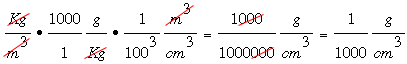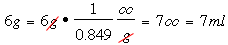SEARCH HOMEMath Central Quandaries & QueriesQuestion from Faiza, a student: I was looking for how to convert grams into mL and I read on this site to convert one to the other you need to know what substance you are measuring. so if I am measuring sugar or any of the baking ingredient like flour how do I convert them from grams to mL is there a simple method I can use to do that?Faiza,

In looking for some information for you I found an interesting page on the Math Forum that essentially says don't do it. If the recipe calls for weight then find a scale and use weights. But this is a school project and maybe you are expected to convert grams to milliliters

There is a site in the UK that I use to find the densities of dry materials. It has densities of some materials in kilograms per cubic meter and you want grams per milliliter of grams per cubic centimeter since 1 milliliter s 1 cubic centimeter. There are 1000 grams in a kilogram and 100 centimeters in a meter so the conversion factor isThus for example the web site I mentioned gives the density of granulated sugar as 849 Kg/m3 so that's 849/1000 = 0.849 g/cc. Thus 6 gm of granulated sugar isPennyMath Central is supported by the University of Regina and The Pacific Institute for the Mathematical Sciences.